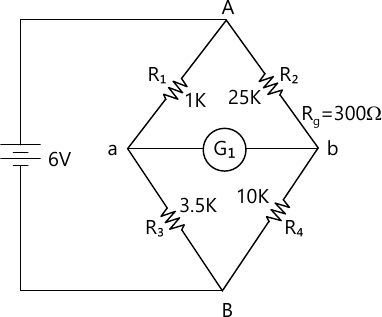MORE IN Electronic Instrumentation
VTU Electronics and Communication Engineering (Semester 3)
Electronic Instrumentation
December 2015
Total marks: --
Total time: --
INSTRUCTIONS
(1) Assume appropriate data and state your reasons
(2) Marks are given to the right of every question
(3) Draw neat diagrams wherever necessary

1 (a) Define following terms as applied to an electronic instruments:
i) Random error
ii) Significant figure
iii) Resolution
6 M
1 (b) Explain the working of a true RMS voltmeter with the help of a suitable block diagram.
8 M
1 (c) A component manufacture constructs certain resistance to be anywhere between 1.14Ω and 1.26 kΩ and classifies them to be 1.2 kΩ resistors. What tolerance be stated? If the resistance values are specified at 25°C and resistor have a temperature coefficient of +500ppm/°C. Calculate the maximum resistance that one of these component might have at 75°C.
6 M

2 (a) Explain working principle of sucessive approximation method of DVM.
8 M
2 (b) With the help of block diagram, explain the operation of measurement of time.
6 M
2 (c) Determine the resolution of a 3 1/2 digit display on IV and 10 V ranges.
6 M

3 (a) Explain working of dual trace CRO.
10 M
3 (b) Explain triggered sweep CRO.
5 M
3 (c) Explain the operation of a electronic switch with the help of a block diagram.
5 M

4 (a) Explain the working of a digital storage oscilloscope and list the advantages of DSO.
10 M
4 (b) Explain the need of time delay in oscilloscopes.
5 M
4 (c) Explain the working of sampling oscilloscope.
5 M

5 (a) Explain principles fixed frequency AF oscillator and variable AF oscillator.
4 M
5 (b) With a neat block diagram, explain sweep frequency generator.
8 M
5 (c) Explain with a neat sketch AF sine and square wave generator.
8 M

6 (a) Explain Maxwell's bridge.
8 M
6 (b) Explain Wagner's earth connection.
6 M
6 (c) An unbalanced Wheatstone bridge is given in Fig. Q6(c), calculate the current through the galvanometer.6 M

7 (a) Explain the construction, principle and operation of LVDT.
8 M
7 (b) Explain resistance thermometer.
6 M
7 (c) Explain thermistor.
6 M

8 (a) Explain LCD with diagram.
6 M
8 (b) Explain power measurement using Bolometer.
8 M
8 (c) Write note on signal conditioning system.
6 M

More question papers from Electronic Instrumentation